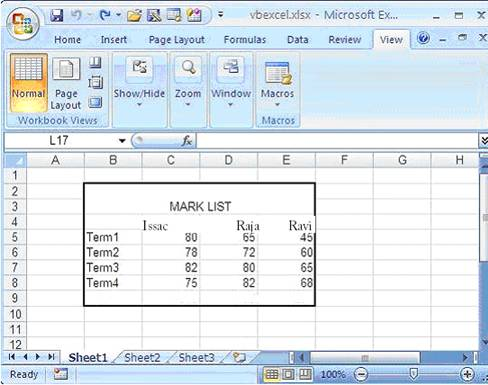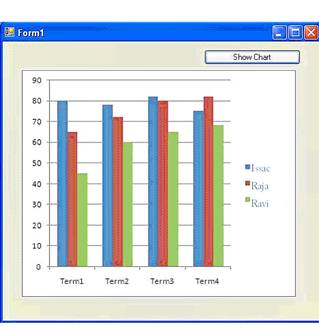# Programming Tutorials

## Using Excel 2007 Chart VB.net Picture Box

By: Issac

The following program shows, how to get the Excel chart as an image in Picture Box .Before we crate a Chart, we have to fill data in Excel sheet. After enter data your Excel sheet is look like the following picture.After filling the data, We have to create a chart object in VB.NET and configure the Chart object with necressary data like positions, size, data range, chart type etc.. And use the command for export chart as picture file, then load the picture from the path to picture box. The following picture shows the program screen after drawing the picture``````
Imports Excel = Microsoft.Office.Interop.Excel
Public Class Form1
Private Sub Button1_Click(ByVal sender As System.Object, _
ByVal e As System.EventArgs) Handles Button1.Click

Dim xlApp As Excel.Application
Dim xlWorkBook As Excel.Workbook
Dim xlWorkSheet As Excel.Worksheet
Dim misValue As Object = System.Reflection.Missing.Value

xlApp = New Excel.ApplicationClass
xlWorkSheet = xlWorkBook.Sheets("sheet1")

xlWorkSheet.Cells(1, 1) = ""
xlWorkSheet.Cells(1, 2) = "Issac"
xlWorkSheet.Cells(1, 3) = "Raja"
xlWorkSheet.Cells(1, 4) = "Ravi"

xlWorkSheet.Cells(2, 1) = "Term1"
xlWorkSheet.Cells(2, 2) = "80"
xlWorkSheet.Cells(2, 3) = "65"
xlWorkSheet.Cells(2, 4) = "45"

xlWorkSheet.Cells(3, 1) = "Term2"
xlWorkSheet.Cells(3, 2) = "78"
xlWorkSheet.Cells(3, 3) = "72"
xlWorkSheet.Cells(3, 4) = "60"

xlWorkSheet.Cells(4, 1) = "Term3"
xlWorkSheet.Cells(4, 2) = "82"
xlWorkSheet.Cells(4, 3) = "80"
xlWorkSheet.Cells(4, 4) = "65"

xlWorkSheet.Cells(5, 1) = "Term4"
xlWorkSheet.Cells(5, 2) = "75"
xlWorkSheet.Cells(5, 3) = "82"
xlWorkSheet.Cells(5, 4) = "68"

'create chart
Dim chartPage As Excel.Chart
Dim xlCharts As Excel.ChartObjects
Dim myChart As Excel.ChartObject
Dim chartRange As Excel.Range

xlCharts = xlWorkSheet.ChartObjects
myChart = xlCharts.Add(10, 80, 300, 250)
chartPage = myChart.Chart
chartRange = xlWorkSheet.Range("A1", "d5")
chartPage.SetSourceData(Source:=chartRange)
chartPage.ChartType = Excel.XlChartType.xlColumnClustered

'exporting chart as picture file
xlWorkSheet.ChartObjects(1).chart.Export(FileName:= _
"C:\excel_chart_export.bmp", FilterName:="BMP")

'load the pipcture into the picture box
PictureBox1.Image = New System.Drawing.Bitmap _
("C:\excel_chart_export.bmp")

xlWorkSheet.SaveAs("C:\vbexcel.xlsx")
xlWorkBook.Close()
xlApp.Quit()

releaseObject(xlApp)
releaseObject(xlWorkBook)
releaseObject(xlWorkSheet)

MsgBox("Chart File Exported !")
End Sub

Private Sub releaseObject(ByVal obj As Object)
Try
System.Runtime.InteropServices.Marshal.ReleaseComObject(obj)
obj = Nothing
Catch ex As Exception
obj = Nothing
Finally
GC.Collect()
End Try
End SubEnd Class``````

When you execute this source code you will get the picture in Picture Box from Excel Chart.

 Most Viewed Articles (in VB.net ) Latest Articles (in VB.net)

Comment on this tutorial

#### Subscribe to Tutorials

1. Thanks Thanks Thanks.....for you
But how

View Tutorial          By: jihad at 2009-02-15 04:48:53

2. Excellent example! Thanks a ton:-)
One ques

View Tutorial          By: Andrew at 2009-04-11 09:15:19

3.
View Tutorial          By: Mudassir at 2009-04-14 07:00:41

4. Thanks for the code.

But I m having

View Tutorial          By: Mudassir at 2009-04-14 07:00:53

5. Thanks for the code!

I got one prob

View Tutorial          By: Mudassir at 2009-04-14 07:34:58

6. Hi,
I need to put in a picturebox on VB .NE

View Tutorial          By: Marcio Rodrigues at 2009-11-27 05:07:09